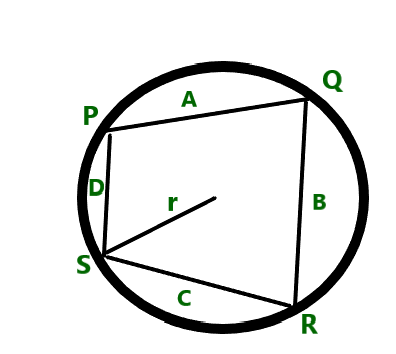Related Articles

# Calculate area of a cyclic quadrilateral with given side lengths

• Difficulty Level : Hard
• Last Updated : 04 Oct, 2021

Given four positive integers A, B, C, and D representing the length of sides of a Cyclic Quadrilateral, the task is to find the area of the Cyclic Quadrilateral.

Examples:

Input: A = 10, B = 15, C = 20, D = 25
Output: 273.861

Input: A = 10, B = 30, C = 50, D = 20
Output: 443.706

Approach: The given problem can be solved based on the following observations:

• A cyclic quadrilateral is a quadrilateral whose vertices all lie on a single circle. The circle is called the circumcircle or circumscribed circle, and the vertices are said to be concyclic.• In the above image above r is the radius of the circumcircle and A, B, C, and D are the lengths of the sides PQ, QR, RS, and SP respectively.
• The area of the quadrilateral is given by Bretschneider’s formula is:where, A, B, C, and D are the sides of the triangle and
α and γ are the opposite angles of the quadrilateral.

Since, the sum of opposite angles of the quadrilateral is 180 degree. Therefore, the value of cos(180/2) = cos(90) = 0.

Therefore, the formula for finding the area reduces to.

Therefore, the idea is to print the value ofas the resultant area of the given quadrilateral.

Below is the implementation of the above approach:

## C++

 // C++ program for the above approach #include using namespace std; // Function to find the area// of cyclic quadrilateralfloat calculateArea(float A, float B,                    float C, float D){    // Stores the value of    // half of the perimeter    float S = (A + B + C + D) / 2;     // Stores area of cyclic quadrilateral    float area = sqrt((S - A) * (S - B)                      * (S - C) * (S - D));     // Return the resultant area    return area;} // Driver Codeint main(){    float A = 10;    float B = 15;    float C = 20;    float D = 25;    cout << calculateArea(A, B, C, D);     return 0;}

## Java

 // Java program for the above approachimport java.io.*; class GFG{     // Function to find the area// of cyclic quadrilateralstatic float calculateArea(float A, float B,                           float C, float D){         // Stores the value of    // half of the perimeter    float S = (A + B + C + D) / 2;     // Stores area of cyclic quadrilateral    float area = (float)Math.sqrt((S - A) * (S - B) *                                  (S - C) * (S - D));     // Return the resultant area    return area;} // Driver codepublic static void main (String[] args){    float A = 10;    float B = 15;    float C = 20;    float D = 25;         System.out.println(calculateArea(A, B, C, D)); }} // This code is contributed by Ankita saini

## Python3

 # Python3 program for the above approachfrom math import sqrt # Function to find the area# of cyclic quadrilateraldef calculateArea(A, B, C, D):         # Stores the value of    # half of the perimeter    S = (A + B + C + D) // 2     # Stores area of cyclic quadrilateral    area = sqrt((S - A) * (S - B) *                (S - C) * (S - D))     # Return the resultant area    return area # Driver Codeif __name__ == '__main__':         A = 10    B = 15    C = 20    D = 25         print(round(calculateArea(A, B, C, D), 3)) # This code is contributed by mohit kumar 29

## C#

 // C# program for the above approachusing System; class GFG{ // Function to find the area// of cyclic quadrilateralstatic float calculateArea(float A, float B,                           float C, float D){         // Stores the value of    // half of the perimeter    float S = (A + B + C + D) / 2;     // Stores area of cyclic quadrilateral    float area = (float)Math.Sqrt((S - A) * (S - B) *                                  (S - C) * (S - D));     // Return the resultant area    return area;} // Driver Codestatic public void Main(){    float A = 10;    float B = 15;    float C = 20;    float D = 25;         Console.Write(calculateArea(A, B, C, D));}} // This code is contributed by code_hunt

## Javascript

 
Output:
273.861

Time Complexity: O(1)
Auxiliary Space: O(1)

Attention reader! Don’t stop learning now. Get hold of all the important DSA concepts with the DSA Self Paced Course at a student-friendly price and become industry ready.  To complete your preparation from learning a language to DS Algo and many more,  please refer Complete Interview Preparation Course.

In case you wish to attend live classes with experts, please refer DSA Live Classes for Working Professionals and Competitive Programming Live for Students.

My Personal Notes arrow_drop_up